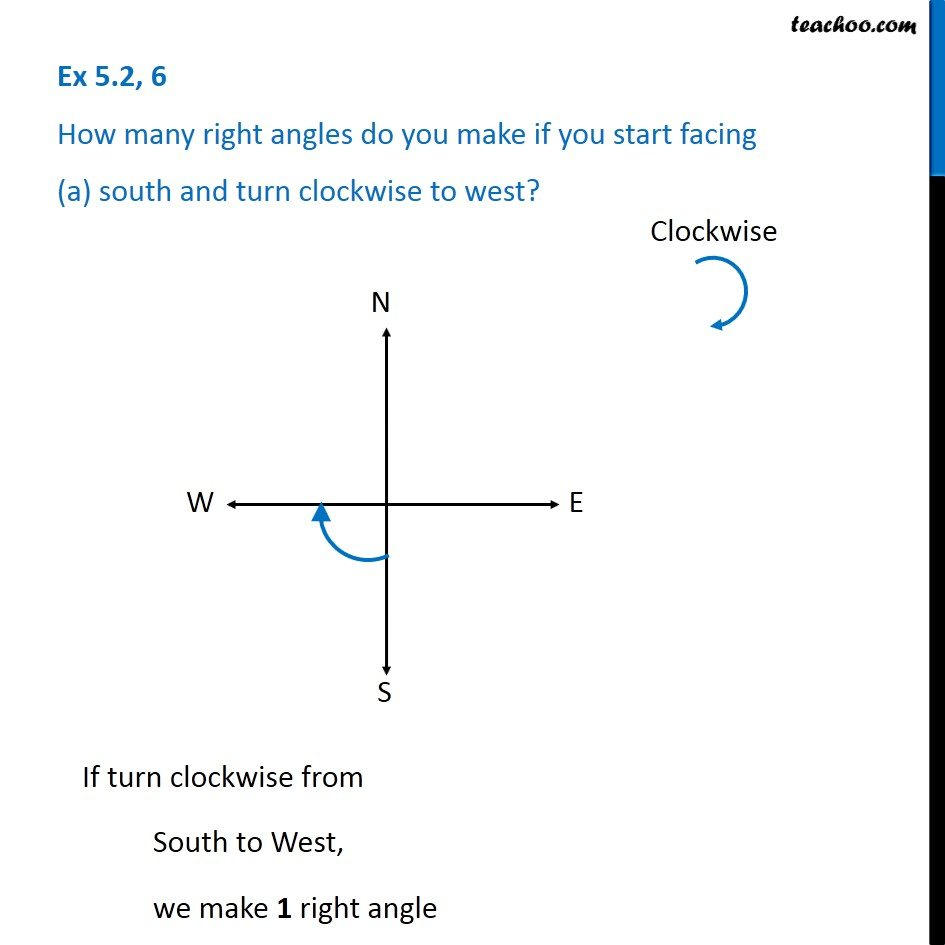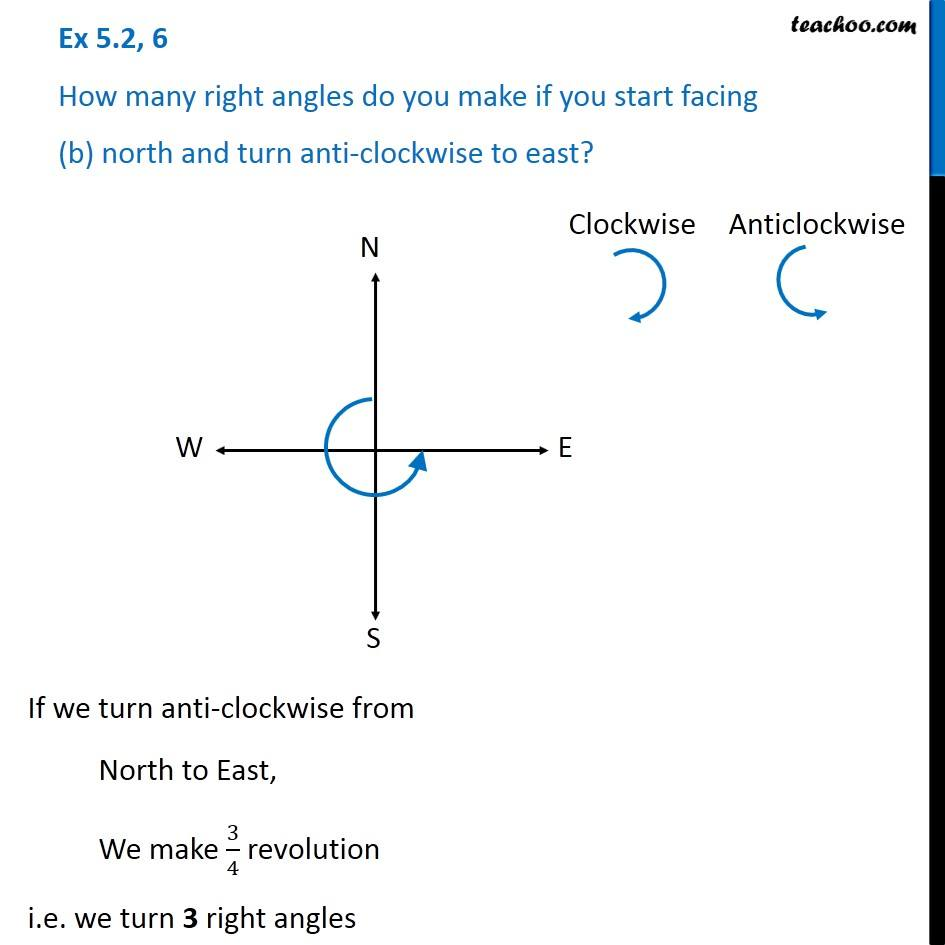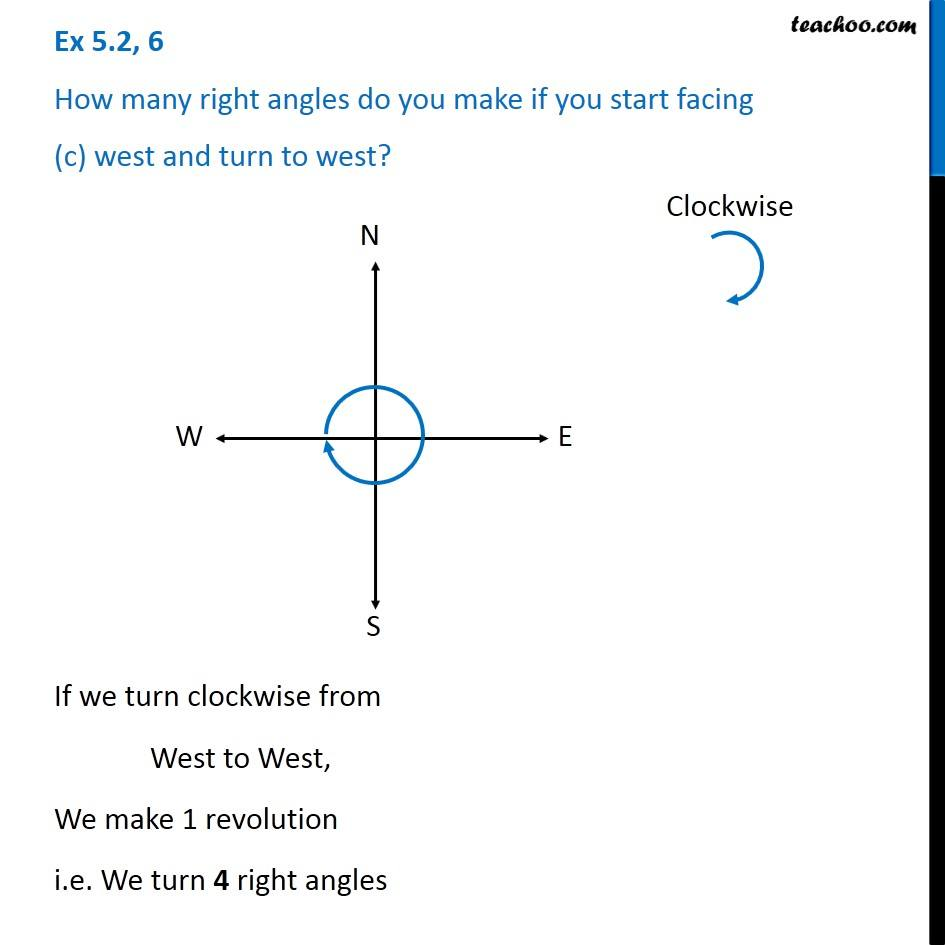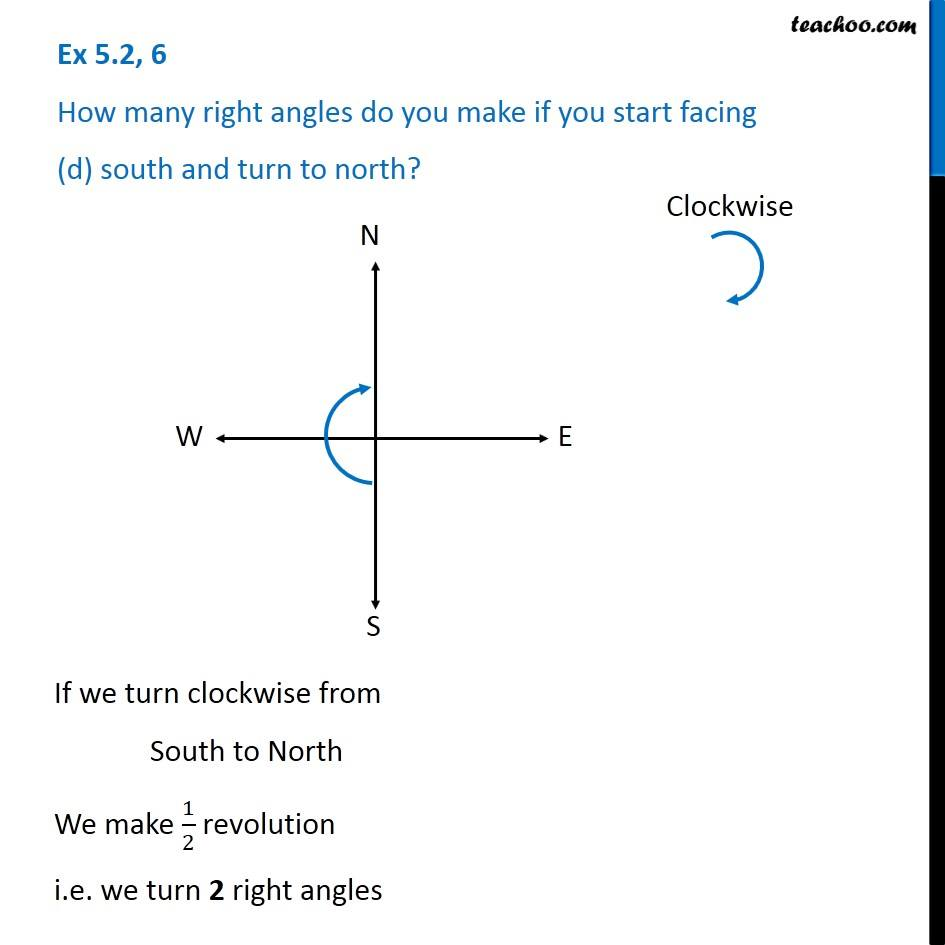Subscribe to our Youtube Channel - https://you.tube/teachoo

1. Chapter 5 Class 6 Understanding Elementary Shapes
2. Concept wise
3. Right angle and straight angle

Transcript

Ex 5.2, 6 How many right angles do you make if you start facing (a) south and turn clockwise to west? If turn clockwise from South to West, we make 1 right angle Ex 5.2, 6 How many right angles do you make if you start facing (b) north and turn anti-clockwise to east? If we turn anti-clockwise from North to East, We make 3/4 revolution i.e. we turn 3 right angles Ex 5.2, 6 How many right angles do you make if you start facing (c) west and turn to west? If we turn clockwise from West to West, We make 1 revolution i.e. We turn 4 right angles Ex 5.2, 6 How many right angles do you make if you start facing (d) south and turn to north? If we turn clockwise from South to North We make 1/2 revolution i.e. we turn 2 right angles

Right angle and straight angle